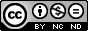## Wednesday, May 28, 2014

### Portfolio Management - A Financial Approach - Part 4

Introduction
We have almost reached the end of our journey through an economic approach to the management of a projects portfolio.

In the first post of this series we introduced some statistical concepts useful to analyze and optimize the composition of a portfolio of projects. In particular, we have characterized projects as black boxes, which absorb resources and generate returns.

In the second post of this series we have derived the concept and mathematical formulation of what is called the efficient frontier, for a simple portfolio made up of two projects.

In the third post we explored a little more in depth the meaning of the the efficient frontier, analyzing its utility and how to use it to manage portfolio in a more effective way.  we sticked to the hypothesis of  a simple 2 projects portfolio as well.

In this post we will remove the hypothesis of  a simple 2 projects portfolio and we will expand what we have seen up to here to a generic portfolio made up of an arbitrary number of projects. We will see what implications this will have on our computational and graphical capabilities.

In the end we will introduce a kind of guide for a step-by-step implementation of the proposed approach.

The N-projects portfolio equations
In Figure 1 we can find the average return and average return standard deviation equations as derived in the second post of this series for the two projects portfolio.Figure 1.

In Figure 2 we can see an extension of these equations for a N-projects portfolio. They are just a little bit more complicated that those depicted in Figure 1 but the rationale remains almost the same. The average return equation is still a linear combination of the average returns of the projects belonging to the portfolio. The average return standard deviation equation is a little bit more complicated, since all the covariances between one project and the others have to be taken into account.
At the end of the post I linked a presentation containing two different notations for the N-projects portfolio equations. These are the notations that we could probably find on math textbooks, but they are absolutely equivalent to the notation in Figure 2.

Clearly, the more projects we add to our portfolio the more a manual evaluation of the equations become unmanageable. Nevertheless, the appeal of this approach lies in the ease of implementation, since the equation presented can be easily implemented in a spreadsheet or in any high level programming language.

Some drawbacks
The first drawback we encounter is that with more than a few project, we completely lose the ability to represent effectively the efficient frontier on a graph. That is why I introduced the topic under the hypothesis of a portfolio made up of just two projects and I have stressed a lot the geometrical approach. At this point, having a clear understanding of the meaning of the equations, a multidimensional extension of the theoretical approach should be straightforward. It should be easy to manage and interpret the presented equations also in a multi-project environment, without a graphical aid.

The second drawback is that the more our portfolio becomes big, the more an evaluation of the efficient frontier's equations become computational intensive. As I have previously said, the method is easy to implement, nevertheless with many projects it quickly becomes computational intensive.
Please remember that the equations in Figure 2 have to be evaluated for each split of the budget between the projects that make up the portfolio. Here comes in our help what we have said in the last post of this series about the quantization of data. We are not dealing with portfolios of securities and we are not allowed to split the budget as we like. Each project could have just a few levels of expenditure and, as a result, the number of equations to be evaluated would fall significantly.

Implementation guide

1. Try to reduce each project to a kind of black box, that is, try to describe it as a function of the absorbed resources (money, people, materials...)  and of the generated benefits (money, services...). Evaluate each project’s average return and return standard deviation, as discussed in the first post of this series.
2. Evaluate the covariance between all the considered projects.
3. Evaluate the efficient frontier's equations for all the possible combination of budget allocations.
4. Select the budget split and the portfolio composition to achieve the desired average return or standard deviation.
5. Pay attention not to be on a unfavorable zone of the efficient frontier, as we have seen in the third post of this series. Unfortunately we cannot afford the luxury of a graphic comparison. So attention must be paid on
• No other available budget allocation can generate a more profitable portfolio composition, in term of greater average return or lower uncertainty. That is, the portfolio composition is not on the red part of the efficient frontier, as shown on Figure 2 of the third post of this series.
• Slightly changing the portfolio composition (also trying combinations that are not actually possible) there is no possibility to achieve an increase of the differential average return greater than the differential uncertainty increase. That is, the portfolio composition is not on the orange part of the efficient frontier, as shown on Figure 3 of the second post of this series.

So, we have reached the end of our journey. I hope you have found this series interesting and useful. Please feel free to contact me for further details, comments, advices...Quest' opera è distribuita con licenza Creative Commons Attribuzione - Non commerciale - Non opere derivate 3.0 Unported.